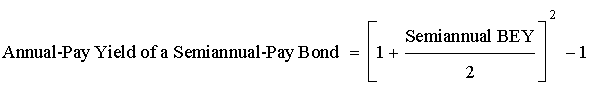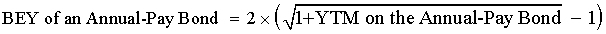Subject 5. Bond Equivalent Yield
Periodic bond yields for both straight and zero-coupon bonds are conventionally computed based on semi-annual periods, as U.S. bonds typically make two coupon payments per year. For example, a zero-coupon bond with a maturity of five years will mature in 10 6-month periods. The periodic yield for that bond, r, is indicated by the equation Price = Maturity value x (1 + r)-10. This yield is an internal rate of return with semi-annual compounding. How do we annualize it?

The convention is to double it and call the result the bond's yield to maturity. This method ignores the effect of compounding semi-annual YTM, and the YTM calculated in this way is called a bond-equivalent yield (BEY).

However, yields of a semi-annual-pay and an annual-pay bond cannot be compared directly without conversion. This conversion can be done in one of the two ways:

• Convert the bond-equivalent yield of a semi-annual-pay bond to an annual-pay bond.• Convert the equivalent annual yield of an annual-pay bond to a bond-equivalent yield.Example
• A Eurobond pays coupon annually. It has an annual-pay YTM of 8%.
• A U.S. corporate bond pays coupon semi-annually. It has a bond equivalent YTM of 7.8%.
• Which bond is more attractive, if all other factors are equal?
Solution 1
• Convert the U.S. corporate bond's bond equivalent yield to an annual-pay yield:
• Annual-pay yield = [1 + 0.078/2]2 - 1 = 7.95% < 8%
• The Eurobond is more attractive since it offers a higher annual-pay yield.
Solution 2
• Convert the Eurobond's annual-pay yield to a bond equivalent yield (BEY):
• BEY = 2 x [(1 + 0.08)0.5 - 1] = 7.85% > 7.8%
• The Eurobond is more attractive since it offers a higher bond equivalent yield.
If you want to change selection, open original toplevel document below and click on "Move attachment"